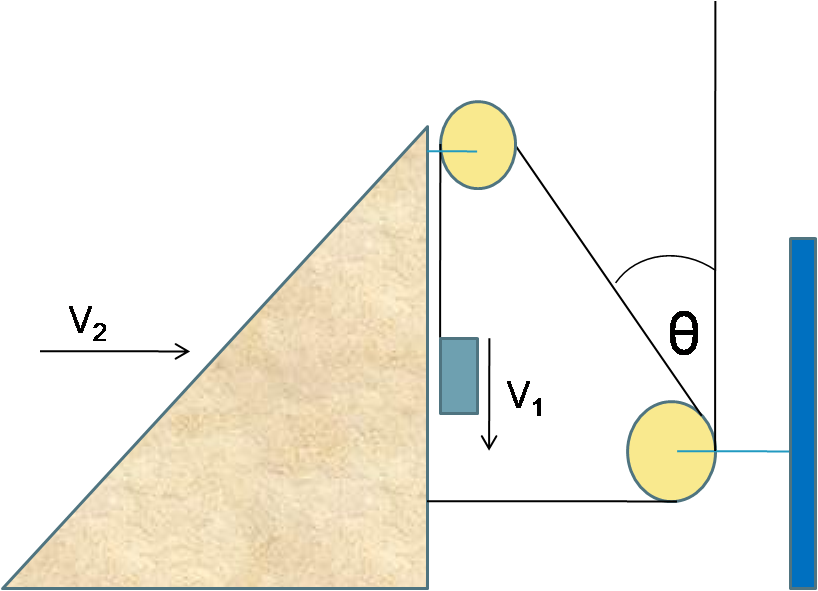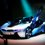# Physics Problem!Find the relation b/w v1 and v2 in terms of v1,v2, and thetaNote by Ajitesh Mishra
6 years, 11 months ago

This discussion board is a place to discuss our Daily Challenges and the math and science related to those challenges. Explanations are more than just a solution — they should explain the steps and thinking strategies that you used to obtain the solution. Comments should further the discussion of math and science.

When posting on Brilliant:

• Use the emojis to react to an explanation, whether you're congratulating a job well done , or just really confused .
• Ask specific questions about the challenge or the steps in somebody's explanation. Well-posed questions can add a lot to the discussion, but posting "I don't understand!" doesn't help anyone.
• Try to contribute something new to the discussion, whether it is an extension, generalization or other idea related to the challenge.

MarkdownAppears as
*italics* or _italics_ italics
**bold** or __bold__ bold
- bulleted- list
• bulleted
• list
1. numbered2. list
1. numbered
2. list
Note: you must add a full line of space before and after lists for them to show up correctly
paragraph 1paragraph 2

paragraph 1

paragraph 2

[example link](https://brilliant.org)example link
> This is a quote
This is a quote
    # I indented these lines
# 4 spaces, and now they show
# up as a code block.

print "hello world"
# I indented these lines
# 4 spaces, and now they show
# up as a code block.

print "hello world"
MathAppears as
Remember to wrap math in $$ ... $$ or $ ... $ to ensure proper formatting.
2 \times 3 $2 \times 3$
2^{34} $2^{34}$
a_{i-1} $a_{i-1}$
\frac{2}{3} $\frac{2}{3}$
\sqrt{2} $\sqrt{2}$
\sum_{i=1}^3 $\sum_{i=1}^3$
\sin \theta $\sin \theta$
\boxed{123} $\boxed{123}$

Sort by:

Let $L$ be the length of the string and $H$ be the height difference between the pulleys.

Let $x$ be the length of the horizontal piece of string and $y$ be the length of the vertical piece of string.

The slanted piece of string has length $L-x-y = \sqrt{x^2+H^2}$. Thus, $(L-x-y)^2 = x^2+H^2$.

Differentiation yields: $2(L-x-y)\left(-\dfrac{dx}{dt}-\dfrac{dy}{dt}\right) = 2x \dfrac{dx}{dt}$.

By definition, $\dfrac{dx}{dt} = -v_2$ and $\dfrac{dy}{dt} = v_1$. Using trigonometry $x = H\tan \theta$ and $L-x-y = H\sec \theta$.

Therefore, $2H\sec\theta \cdot (v_2 - v_1) = 2H\tan\theta \cdot (-v_2)$. Solving yields $v_1 = v_2(1+\sin \theta)$.

- 6 years, 11 months ago

Awesome Solution Dude...!!!

- 6 years, 11 months ago

Wow! Good job Jimmy!

- 6 years, 11 months ago

i think $v_1$=$v_2$$cos\theta$ Not pretty sure but still..i think..

- 6 years, 11 months ago

no .. it isn't the right ans

- 6 years, 11 months ago

use the concept that length on the thread remains constant. :)

- 6 years, 11 months ago

Does $\theta$ stay constant? My guess is no, but I can't tell for certain from the diagram.

- 6 years, 11 months ago

Yes , $\theta$ will definitely not remain the same . $\theta$ would gradually decrease .

- 6 years, 11 months ago

won't it also be in terms of the masses of the two objects? (determining their acceleration from the tension in the thread and gravity)

- 6 years, 11 months ago

nope ..masses aren't needed..

- 6 years, 11 months ago

is the velocity v1 with respect to wedge or with respect to ground?

- 6 years, 11 months ago

$v_2(1+\sin\theta)=v_1\sin\theta$

- 6 years, 11 months ago

v1/(1+sinθ)=v2 I think so!!!

- 6 years, 11 months ago

concept behind the problem is use of constraint motion

- 6 years, 11 months ago

xdx=ldL sinθ=dL/dx sinθ+1=(dL+dx)/dx sinθ+1=V1/V2

- 6 years, 11 months ago

constraint relations

- 6 years, 11 months ago

Another way to derive the relation is by using the fact that work done by tension is zero (by newton's third law). We get $\sum$T.s=0 . Differentiating we get, $\sum$T.v=0

- 6 years, 11 months ago

using the virtual work method..-T(1+sin(thetha))x2 + Tx1=0. Differentiating this constraint equation we get the relation v2(1+sin(thetha))=v1. (x2 is wedge's displacement and x1 is the block's displacement)

- 6 years, 11 months ago

My guess... it's "v1 = v2 + v2*sin(theta)". Is that right?

- 6 years, 11 months ago

is it a guess or something else??

- 6 years, 11 months ago

More than a guess. I have used logic but might be wrong.

- 6 years, 11 months ago

by constrain relation,v2=v1tan theta

- 6 years, 11 months ago